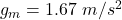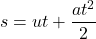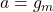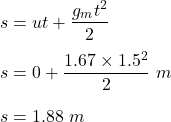## An astronaut on the moon drops a feather from rest (v = 0). The acceleration due to gravity is 1.67 m/s​ 2​ . If the feather begins 2 meters

Question

An astronaut on the moon drops a feather from rest (v = 0). The acceleration due to gravity is 1.67 m/s​ 2​ . If the feather begins 2 meters above the moon’s surface, what will be its final position after falling for 1.5 seconds?

in progress 0
2 months 2021-07-21T10:11:53+00:00 1 Answers 2 views 0

1. Given :

Initial velocity , u = 0 m/s .

Acceleration due to gravity on moon ,.

Height , h = 2 m .

To Find :

Final position after falling for 1.5 seconds .

Solution :

We know , by equation of motion :Here ,.

So , equation will transform by :Therefore , the height form moon’s surface is 1.88 m .

Hence , this is the required solution .